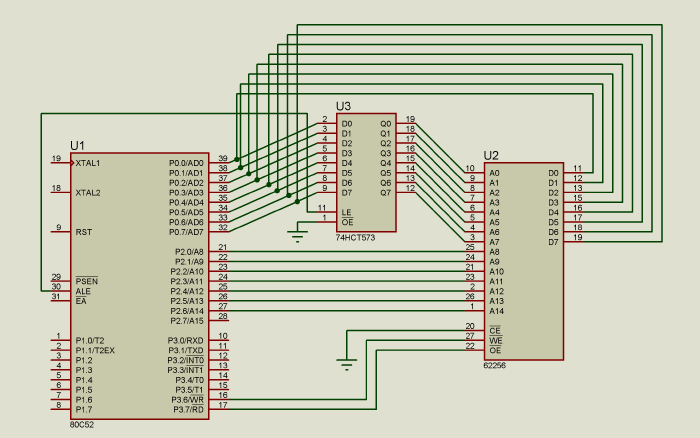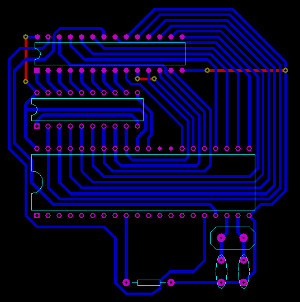# Gzalo.com

## External RAM memories in MCS51 architecture (8051/8052)

When an external memory position is read (movx a, @dptr), the 8052 does the following:

1. P0 = DPL
2. Pulses ALE terminal
3. P2 = DPH
4. Pulses P3_7 (RD) terminal
5. A = P0

When writing to external memory (movx @dptr, a), the 8052 does something similar:

1. P0 = DPL
2. Pulses ALE terminal
3. P2 = DPH
4. P0 = A
5. Pulses P3_6 (WR) terminal

Because P0 is used as the data bus and as the low byte of the address bus (data and address multiplexing), an 8 bit latch is needed in order to store the lower half of the address.

The circuit is then the following:Because the memory allows random access, it doesn't matter if address bus terminals are exchanged, as long as they are all connected it will work. The same thing happens with the data line. As long as the IC is a RAM memory. If it was an EEPROM it would be different, because it might need to be programmed in another circuit, and then the addresses would be mixed up.

That allows us to do the PCB using a single layer with only a few wire bridges, as the image shows:## Optimized way of cleaning a buffer in external memory

Let's assume that there is a 1024 byte buffer, in external memory, address 0, in a 8052 microcontroller running at 24 MHz, 2 MIPS.

The easier way to clean that buffer would be to use four loops, each one cleaning 256 bytes of the external memory.

``````
mov dptr, #0  ;Starting address of the buffer
mov a, #0xAA  ;Value to set the buffer with

mov r0, #0
lp1:movx @dptr, a
inc dptr
djnz r0, lp1

mov r0, #0
lp2:movx @dptr, a
inc dptr
djnz r0, lp2

mov r0, #0
lp3:movx @dptr, a
inc dptr
djnz r0, lp3

mov r0, #0
lp4:movx @dptr, a
inc dptr
djnz r0, lp4
``````

This solution takes 3076.5 uS (measured with Keil uVision simulator).

For some critical applications, this may be a bit too much, so an optimization might be needed.

The first optimization is to remove the four loops and replace it with just one:

``````
mov    dptr, #0
mov a, #0xAA

mov r0, #0
lp1:movx @dptr, a
inc    dptr
movx @dptr, a
inc    dptr
movx @dptr, a
inc    dptr
movx @dptr, a
inc    dptr
djnz r0, lp1
``````

Besides reducing the code size, it also reduces the execution time, to 2307uS, a 30% improvement.

In order to reduce it further, a instruction movx @r0, a, can be used, which does the same as DPTR but with only an 8 bit register, using P2 as the higher byte of the address

``````
mov a, #0xAA
mov r0, #0
lp1:
mov p2, #0
movx @r0, a
inc p2
movx @r0, a
inc p2
movx @r0, a
inc p2
movx @r0, a
djnz r0, lp1
``````

Now it only takes 1922 uS.

By unrolling the loop (reducing the frequency between djnz by adding more instructions that affect data) a time of 1858uS (x2) or 1826 (x4) is achievable.

This methods uses only 1603uS! Almost half the time of the first one!

``````
mov a, #0xAA
mov r0, #0
mov p2, #0
lp1:
movx @r0, a  ;P2 = 0
inc p2

movx @r0, a  ;P2 = 1
inc p2

movx @r0, a  ;P2 = 2
inc p2

movx @r0, a  ;P2 = 3
dec r0

movx @r0, a  ;P2 = 3
dec p2

movx @r0, a  ;P2 = 2
dec p2

movx @r0, a  ;P2 = 1
dec p2

movx @r0, a  ;P2 = 0

djnz r0, lp1
``````

In order to analyze the absolute efficiency, a simple analysis can be done: movx take 1uS, and inc 0.5uS, so in order to be 100% efficient it should take 1536 uS to clean the buffer. Considering that the method takes 1603 uS, its 93% efficient, quite high for using just some simple unroll optimizations.

The idea of optimization is to find a balance between extra memory usage (code and data) and the algorithm complexity and speed.